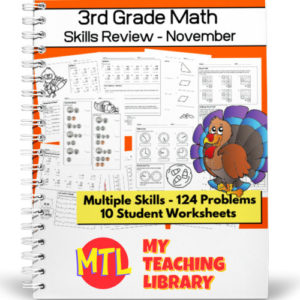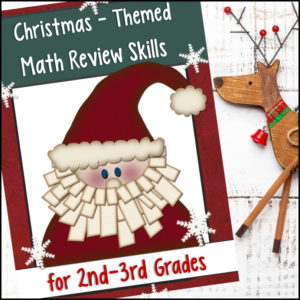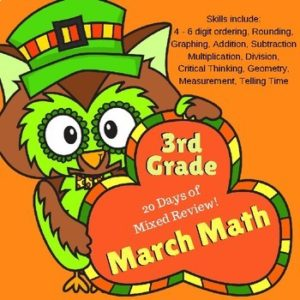Showing all 18 results

•\$2.50

Math Skills Practice Workbook has been designed to review and practice 3rd grade skills. These skills include addition, subtraction, money, comparing numbers, telling time, and identifying polygons.

Includes 10 student worksheets + answer keys

• Sale!\$6.00 \$5.00

This resource will allow students to practice converting Roman numerals as well as comparing Roman numerals to Arabic numbers! There are 20 student worksheets with 240 total questions.

Use this bundle if you need worksheets for differing levels of knowledge:

• Roman Numerals 1 to 99 (I, V, X, L)
• Roman Numerals 1 – 1600 (I, V, X, L, C, D, M)

For a fun, holiday themed twist, each worksheet as a Thanksgiving themed maze!

This product contains two MTL resources:

•\$3.00

Give students the practice they need with Roman Numerals! Students will be asked to convert numerals from 1 to 99. They will convert from Arabic to Roman and Roman to Arabic. They will also be asked to compare Roman numerals with Arabic numbers by placing the correct sign: greater than, less than or equal. Includes 10 worksheets – 120 problems:

• 3 worksheets converting Arabic numbers to Roman numerals
• 4 worksheets converting Roman numerals to Arabic numbers
• 3 worksheets comparing Roman numerals with Arabic numbers (<, >, =)
•\$2.50

Here are 10 Skip Counting Worksheets for students to practice skip counting. Students will practice skip counting both up and down. This resource includes 10 worksheets, each with 10 problems:

• 4 worksheets – counting by twos
• 3 worksheets – counting by fives
• 3 worksheets – counting by tens

Each worksheet also includes a fun Thanksgiving themed maze. Perfect to use in November or really anytime throughout the year!

•\$4.99

OVER 1,800 problems, your students will have the practice they need to understand and learn their multiplication facts 0 to 10!

The resource begins with repeated addition (to reinforce the concept of addition in relation to multiplication) and ends with students completing all multiplication facts.

•\$2.00

Give students the practice they need to master beginning long division with these 10 worksheets (160 problems) resource. Students will solve division problems with 2 digit dividends and 1 digit quotients. (No remainders) – Answer Keys provided

•\$6.99

This download contains 160 student pages PACKED with 2nd Grade learning! In it, you’ll find a variety of fun and engaging worksheets, puzzles and activities that will allow students to learn, practice and review needed skills: 1, 2 and 3 digit Addition and Subtraction, Principles of Multiplication, Multiplication x2, Number Sense, Greater than / Less than, Fractions, Shapes, Patterns, Money, Writing, Rhyming, Classification, Phonics, Syllables, Reading, Sight Words and more!

•\$8.00

Christmas Math for 2nd and 3rd Grades is a large download with 76 student pages! Great for math centers, seat work, cooperative learning and/or fun holiday related homework. (Although this unit was designed for 2nd and 3rd grades, it would work wonderful for advanced 1st grade or remedial 4th grade!)

•\$3.00

Help students learn and practice the basic concept behind multiplication with these 280 visual model problems. Designed for 2nd – 3rd Grades, students will demonstrate the ability to…

• – Determine the quantity of shapes shown
• – Create equations from arrays
• – Create arrays from given equations

Why arrays? A multiplication array is simply an arrangement of rows or columns that matches a multiplication equation. Students can more readily develop an understanding of multiplication concepts when they see visual representations of the computation process. Arrays are also the easiest and most logical way to show kids the commutative property of multiplication.

Included: 30 worksheets + answer keys.

•\$5.00

Workbook filled with 2nd Grade skills – Over 170 pages.

•\$3.00

Designed to help your 3rd grade students practice and reinforce math skills including 4 – 6 digit ordering least to greatest; rounding to the nearest ten, hundred, thousand graphing; addition; subtraction; multiplication, division, critical thinking, geometry (area), measurement (quantity), telling time. Includes 20 fun activity pages.

•\$2.00

Help students learn and understand the basics of division through visual models (division arrays). This resource includes 5 worksheets (8 problems on each) plus answer keys.

•\$5.00

This skills workbook has been designed for 2nd Grade (160+ pages).

•\$5.00

March Math Skills Review for 2nd-3rd Grades is a NO PREP, St. Patrick’s Day themed packet and is packed full of engaging activities to keep kids learning and reviewing skills during the month of March!

NO PREP means…you do not need to laminate, prep or use any color ink. These are simply PRINT & GO pages.

•\$2.50

•\$3.00

This resource will help your students practice and reinforce important 2nd Grade Math skills including: rounding, graphing, adding, subtracting, fractions, money, patterns, measurement, telling time, using critical thinking skills and more. Includes word problems! Your students will love these St. Patrick’s Day – Shamrock themed pages.

•\$4.00

This resource includes 77 student worksheets designed to cover a multitude of 2nd-3rd grade math skills such as:

• – Addition & subtraction (with and without carrying / borrowing)
• – Word problems
• – Critical thinking skills
• – Find the missing addend or subtrahend
• – Odd & Even numbers
• – Roman Numerals
• – Order of operations
•  – Expanded form
• – Skip counting (by 50, 100, 250)
• – Rounding (nearest 10, 100)
• – Telling time
• – Counting money
• – Fractions (equivalent, comparing)
• – Multiplication & Division
• – Measurement
• – Shapes (2D & 3D)
• – Early Geometry (faces, edges, vertices, perimeter)
•\$5.00

This Winter resource is PRINT and GO – Ready to use and with over 70 student pages can be used throughout the Winter! Perfect for morning work, math centers, homework folders, skill reinforcement and sub-folders.